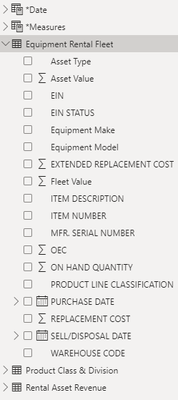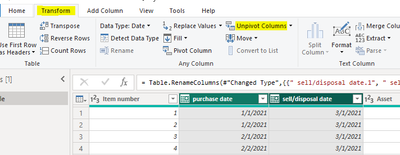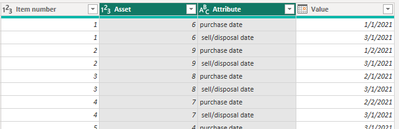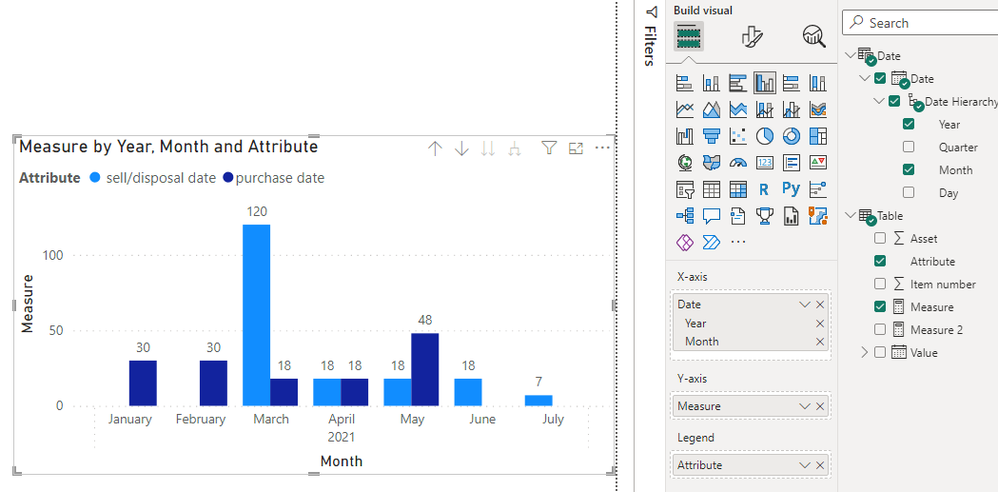cancel
Showing results for
Did you mean:## Asset Value Timeline Visualization

I have a table that contains asset information such as Item Number, Fleet Value (Asset * Qty), purchase date and sell/disposal date. I am looking for some help in creating a visual to show sum(fleet value) by month. Do you guys have any ideas on how to relate an aggregate fleet value between months of being purchased and sold?2 ACCEPTED SOLUTIONSCommunity Support

Hi  @burtta ,

I created some data:Here are the steps you can follow：

1. Select [purchase date] and [sell/disposal date] --  Transform – Unpivot Columns.Result:2. Create calaculated table.

``````Date =
CALENDAR(
DATE(
2021,1,1),
DATE(
2021,12,31))``````3. Create measure.

``````Measure =
var _Qty=
COUNTX(
FILTER(ALL('Table'),
'Table'[Attribute]=MAX('Table'[Attribute])&&
YEAR('Table'[Value])=YEAR(MAX('Date'[Date]))&&
MONTH('Table'[Value])=MONTH(MAX('Date'[Date]))),[Item number])
var _sum=
SUMX(
FILTER(ALL('Table'),
'Table'[Attribute]=MAX('Table'[Attribute])&&
YEAR('Table'[Value])=YEAR(MAX('Date'[Date]))&&
MONTH('Table'[Value])=MONTH(MAX('Date'[Date]))),[Asset])
return
_sum * _Qty``````

4. Result:Best Regards,

Liu Yang

If this post helps, then please consider Accept it as the solution to help the other members find it more quicklyHere is the measure that resolved my problem.

Prev12MoRevExt (Fleet) =
CALCULATE(
SUM('Rental Asset Revenue'[Revenue EXT]),
CALCULATETABLE(
'*Date',
FILTER(
'*Date',
'*Date'[Date] >=
DATE(YEAR(TODAY()) - 1, 5, 1)
&&
'*Date'[Date] <=
EOMONTH(
TODAY(),
-1
)
)
)
)
3 REPLIES 3Here is the measure that resolved my problem.

Prev12MoRevExt (Fleet) =
CALCULATE(
SUM('Rental Asset Revenue'[Revenue EXT]),
CALCULATETABLE(
'*Date',
FILTER(
'*Date',
'*Date'[Date] >=
DATE(YEAR(TODAY()) - 1, 5, 1)
&&
'*Date'[Date] <=
EOMONTH(
TODAY(),
-1
)
)
)
)Super User

Hi,

Share some sample data and show the expected result.

Regards,
Ashish Mathur
http://www.ashishmathur.comCommunity Support

Hi  @burtta ,

I created some data:Here are the steps you can follow：

1. Select [purchase date] and [sell/disposal date] --  Transform – Unpivot Columns.Result:2. Create calaculated table.

``````Date =
CALENDAR(
DATE(
2021,1,1),
DATE(
2021,12,31))``````3. Create measure.

``````Measure =
var _Qty=
COUNTX(
FILTER(ALL('Table'),
'Table'[Attribute]=MAX('Table'[Attribute])&&
YEAR('Table'[Value])=YEAR(MAX('Date'[Date]))&&
MONTH('Table'[Value])=MONTH(MAX('Date'[Date]))),[Item number])
var _sum=
SUMX(
FILTER(ALL('Table'),
'Table'[Attribute]=MAX('Table'[Attribute])&&
YEAR('Table'[Value])=YEAR(MAX('Date'[Date]))&&
MONTH('Table'[Value])=MONTH(MAX('Date'[Date]))),[Asset])
return
_sum * _Qty``````

4. Result:Best Regards,

Liu Yang

If this post helps, then please consider Accept it as the solution to help the other members find it more quickly